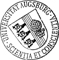Domain Decomposition and Balanced Truncation Model Reduction for Shape Optimization of the Stokes System

• The optimal design of structures and systems described by partial differential equations (PDEs) often gives rise to large-scale optimization problems, in particular if the underlying system of PDEs represents a multi-scale, multi-physics problem. Therefore, reduced order modeling techniques such as balanced truncation model reduction, proper orthogonal decomposition, or reduced basis methods are used to significantly decrease the computational complexity while maintaining the desired accuracy of the approximation. In this paper, we are interested in such shape optimization problems where the design issue is restricted to a relatively small portion of the computational domain. In this case, it appears to be natural to rely on a full order model only in that specific part of the domain and to use a reduced order model elsewhere. A convenient methodology to realize this idea consists in a suitable combination of domain decomposition techniques and balanced truncation model reduction. WeThe optimal design of structures and systems described by partial differential equations (PDEs) often gives rise to large-scale optimization problems, in particular if the underlying system of PDEs represents a multi-scale, multi-physics problem. Therefore, reduced order modeling techniques such as balanced truncation model reduction, proper orthogonal decomposition, or reduced basis methods are used to significantly decrease the computational complexity while maintaining the desired accuracy of the approximation. In this paper, we are interested in such shape optimization problems where the design issue is restricted to a relatively small portion of the computational domain. In this case, it appears to be natural to rely on a full order model only in that specific part of the domain and to use a reduced order model elsewhere. A convenient methodology to realize this idea consists in a suitable combination of domain decomposition techniques and balanced truncation model reduction. We will consider such an approach for shape optimization problems associated with the time-dependent Stokes system and derive explicit error bounds for the modeling error. As an application in life sciences, we will be concerned with the optimal design of capillary barriers as part of a network of microchannels and reservoirs on microfluidic biochips that are used in clinical diagnostics, pharmacology, and forensics for high-throughput screening and hybridization in genomics and protein profiling in proteomics.• Dokument_1.pdfAuthor: Harbir AntilGND, Matthias Heinkenschloss, Ronald H. W. HoppeGND urn:nbn:de:bvb:384-opus4-10780 https://opus.bibliothek.uni-augsburg.de/opus4/1278 Preprints des Instituts für Mathematik der Universität Augsburg (2009-17) Preprint English Universität Augsburg University of Houston, Rice University 2009/07/13 shape optimization; domain decomposition; balanced truncation model reduction; Stokes system Gestaltoptimierung; Partielle Differentialgleichung; Gebietszerlegungsmethode Mathematisch-Naturwissenschaftlich-Technische Fakultät Mathematisch-Naturwissenschaftlich-Technische Fakultät / Institut für Mathematik Mathematisch-Naturwissenschaftlich-Technische Fakultät / Institut für Mathematik / Lehrstuhl für Numerische Mathematik 5 Naturwissenschaften und Mathematik / 51 Mathematik / 510 MathematikDeutsches Urheberrecht mit Print on Demand